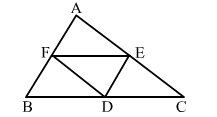# Show that the quadrilateral formed by joining the midpoints of the pairs of adjacent sides of a rectangle is a rhombus.

Question:

Show that the quadrilateral formed by joining the midpoints of the pairs of adjacent sides of a rectangle is a rhombus.Solution:

Let ABCD be the rectangle and P, Q, R and S be the midpoints of AB, BC, CD and DA, respectively.
Join AC, a diagonal of the rectangle.
In ∆ ABC, we have:

$\therefore P Q \| A C$ and $P Q=\frac{1}{2} A C$   [By midpoint theorem]

Again, in ∆ DACthe points S and R are the mid points of AD and DC, respectively.

$\therefore S R \| A C$ and $S R=\frac{1}{2} A C$     [By midpoint theorem]

Now, PQ ∣∣ AC and SR ∣∣ AC
⇒ PQ ∣∣ SR

Also, PQ = SR   [Each equal to $\frac{1}{2} A C$ ]        ........ (i)

So, PQRS is a parallelogram.
Now, in ∆SAP and QBP, we have:
AS = BQ
A = ∠B = 90o
AP = BP
i.e., ∆SAP ≅ ∆QBP​
∴ PS = PQ                ...(ii)
Similarly, ∆SDR ≅ ∆QCR​
∴ SR = RQ                ...(iii)
From (i), (ii) and (iii), we have:
PQ = PQ = SR = RQ
Hence, PQRS is a rhombus.